GAMMA RAY LOG BASICS
The first gamma ray logs were run by Lane Wells in 1936. It looked similar to an SP log and was easy to use in correlating zones from well to well. It was hailed as a great advance over the SP log because its value does not depend on mud or formation water resistivity.

Many elements are naturally radioactive as a result of basic particle physics. Gamma ray logs measures the number of natural gamma rays emitted by the rocks surrounding the tool. This is often proportional to the amount of shale in the rocks, but there are other causes of gamma radiation. The spectral gamma ray log breaks up the total gamma ray response into three components, namely those due to potassium, thorium, and uranium. These measurements are used to distinguish the mineralogy in a shale or other radioactive minerals.

The log can be run in air or mud filled open holes, and also in cased holes, although the response is attenuated by the cement and pipe thickness.

References:
1. Gamma Ray Well Logging,
L.G. Howell, A Forsch, Geophysics, 1939.

2. Gamma Ray Well Logging,
F.P. Kokesh, Oil and Gas Journal, 1951.

3. Shaly Sand Evaluation Using Gamma Ray Spectrometry,
G. Marett, P. Chevalier, P.Souhuite, J. Suau, SPWLA, 1976.UNITS OF MEASUREMENT

In the early days of the logging industry, gamma ray flux was recorded in micrograms Radium equivalent per ton (ug-Ra equiv / ton) prior to about 1960. After that time, logs were calibrated in API units based on known radiation levels of artificial formations in test pits located in Houston. The usual scale for old style logs was 0 to 10 ug Ra and 0 to 100, 0 to 120, or 0 to 150 API units for newer logs. There is an exact conversion between ug-Ra and API units, but since the old logging tools were rarely calibrated, this conversion is seldom useful. The pragmatic solution is to multiply ug-Ra by 10 to obtain an approximate API units scale.STATISTICAL VARIATIONS
Radiation is naturally erratic. A stationary detector facing a given gamma ray flux will not see a constant stream of gamma rays. To obtain a reliable count rate, measuring instruments record the total number of emissions over a period of time, known as the time constant. For most gamma ray tools, the time constant is 1 or 2 seconds to obtain a smooth log curve. The differences in count rates between one time constant and another are called statistical variations.GAMMA RAY TOOL RESPONSE
An empirical relationship between potassium content and gamma ray API units is reproduced below for the standard gamma ray logging conditions of 8" borehole, 10 lb/gal mud and 3 5/8" scintillation NaI detector type tool. This relationship was originally developed by the author while calibrating gamma ray log response to potash content of potash (sylvite and carnallite) beds in 1963. For other borehole environments refer to appropriate borehole correction charts.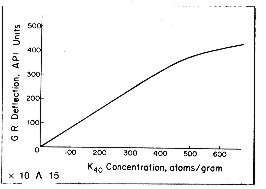The flattening effect at high count rates is due to the dead time of the detector system. Dead time is the time it takes to transmit the recorded pulse to the surface. For other tool types, with different detectors and dead times, the relationship must be found by calibration. Newer tools (post 1980) have a linear response up to 1000 API units.

Special purpose gamma ray tools, such as those used by USGS in mineral investigations, are not calibrated to oil field standards. Conversion to oil field or mineral values will require calibration on a project-by-project basis.GAMMA RAY TOOL CALIBRATION
A prototype tool is at some time placed in a test well with a calibrating formation with a known count gamma ray rate, based on the API standard test well in Houston. This well has an artificial formation with an 8 inch borehole and a radioactivity level designated as 200 API units. The actual count rate of a tool in that test hole is used to obtain the number of counts per second equivalent to a given number of  API Units. The equation would be GRapi = A * CPS where A is the number of API  Units per cps.

But detectors age, and tool sensitivity varies, so A is not constant over time and we need a secondary calibrator, namely a jig with a near-constant GR source. Still at the test pit site and immediately after finding the sensitivity constant A, we place the jig a fixed distance from the tool and note how many cps it adds to the local background radiation. Since we know A for this tool at this moment, we can determine the number of API units that the jig represents at that distance from the tool. Suppose this jig adds 200 API Units to the background while at the test pit. The equation is GRapi = 200 + BKGapi. BKGapi is the GR background in API units. However BKGapi is unknown, but could be estimate from BKGapi = A * BKGcps, or any other arbitrary value.

This is not a great method because we don't know the background radiation level in API units (only in CPS). So the process in the field is iterative and imprecise.

EXAMPLE:
On arrival at the wellsite, the logging tool is powered up on the catwalk. The background gamma radiation is noted: suppose GRbkg = 60 (uncalibrated) units. Apply a 200 API jig and observe the tool response: suppose GR200 = 290 units. The difference between GR200 and GRbkg = 290 - 60 = 230 (not the 200 API units that the jig represents). The error is 30 units and the percent error is 30/290 = 10%. Reduce tool sensitivity by 10% giving GRbkg = 60 - 6 = 54, and GR200 = 290 - 29 = 261. The difference is now only 261 - 54 = 7 units. Reduce sensitivity again, by about 2%, giving 255 - 53 = 202 Units- A tiny tweak to lower the sensitivity will finish the calibration.

For a two point calibration, we determine the difference in count rates caused by placing the jig at two known distances from the tool.
1: (GR1api - GR2api) = C * (CPS1 - CPS2)
2: C = GRapi difference / CPS difference
and 3: GRlog = C * CPS

EXAMPLE: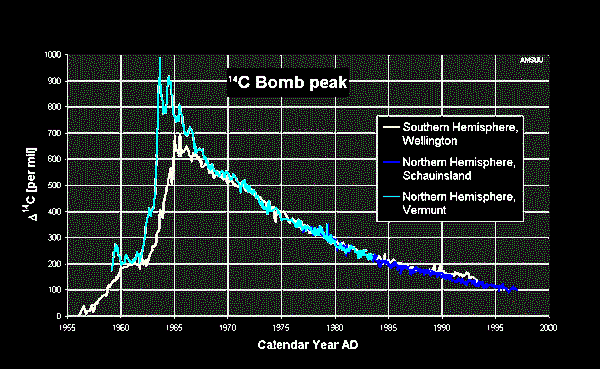The GRapi difference for a typical 2 point jig is 160 API units. At the wellsite, apply the jig in position 1, record the CPS reading, change to jig position 2, and read the CPS value. Then  C = 160 / (CPS1 - CPS2) and GRlog = C * CPSlog and no background gamma ray reading is needed.

Note that in the 1960's and 70's the GR background was very high due to bomb tests by USA and Russia. Background did not reduce to near normal until the mid to late 1980's. The GR calibration records in that era were recorded on the field prints but were removed prior to preparation of the final log prints. The graph at the right might explain why cancer rates are so high for those born in the A-Bomb era.SPECTRAL GAMMA RAY LOGS
In gamma ray spectral logging, the three main gamma ray contributors, potassium, thorium, and uranium, give gamma rays of different energy levels. By appropriate filtering, the total gamma ray flux can be separated into the three components. This aids log analysis as thorium is a good shale indicator when uranium masks the total GR response. Thorium-potassium ratio and other combinations of curves can be used for mineral identification and clay typing. Finally, uranium counts can be subtracted from the total counts to give a uranium corrected gamma ray curve that is easier to use and to correlate from well to well.

The natural  gamma ray logging tools provide increased detection efficiency with spectral processing to significantly improve measurement precision and reduce environmental corrections. Sensitivity to the barite content of mud is eliminated by using only the high-energy gamma rays for analysis. Real-time corrections are made for borehole size and the borehole potassium contribution. These corrections were not made on older logs (pre-200?) so be aware.

Log scales may vary but uranium and thorium are usually scale in parts per million (ppm) and potassium in percent. Curve names may also vary but POTA, URAN, and THOR are common.

Although total gamma ray is also presented on the log in API units, it is sometimes useful to recalculate the total GR from the elemental GR breakdown:

1: GRtotal = 4 * THOR + 8 * URAN + 16 * POTA

Where:  URAN and THOR are ppm and POTA is in %. GRtotal is in API units.

If uranium is known in ppm, total gamma ray can be corrected for uranium with:
2: CGR = GRtotal - 8 * URAN

This makes it easier to use the GR as a shale indicator, especially in unconventional (gas shale) reservoirs.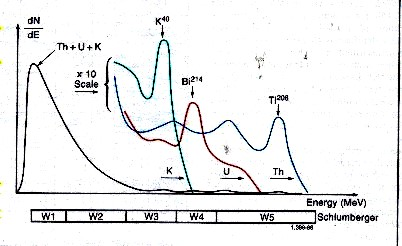Spectral breakdown of total GR into its three major components.

Gamma rays emitted by the rocks rarely reach the detector directly. Instead, they are scattered and lose energy through three possible interactions with the formation; the photoelectric effect, Compton scattering, and pair production. Because of these interactions and the response of the sodium iodide scintillation detector, the spectra are degraded to the rather “smeared” spectra shown above.

The low-energy part of the detected spectrum is divided into two energy windows, W1 and W2 which are used to determine total GR counts.

The high-energy part of the spectrum is divided into three energy windows, W3 (potassium), W4 (uranium), and W5 (thorium) covering a characteristic peak of the three radioactivity series. Knowing the response of the tool and the number of counts in each window, it is possible to determine the amounts of thorium 232, uranium 238, and potassium 40 in the formation. There are relatively few counts in the high-energy range where peak discrimination is best; therefore, measurements are subject to large statistical variations, even at low logging speeds.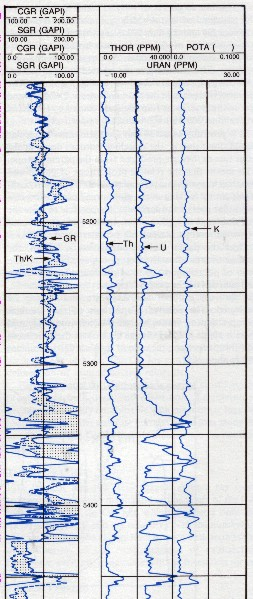Gamma Ray Spectral Log Presentation. Note difference between standard gamma ray (SGR) and uranium corrected gamma ray (CGR).

By including a contribution from the high-count rate, low-energy part of the spectrum (Windows W4 and W5), these high statistical variations in the high-energy windows can be reduced by a factor of 1.5 to 2. The statistics are further reduced by another factor of 1.5 to 2 by using a filtering technique that compares the counts at a particular depth with the previous values in such a way that spurious changes are eliminated while the effects of formation changes are retained.GAMMA RAY LOG CURVE NAMESGamma Ray Log (GR)
 Curves Units Abbreviations gamma ray api GR or SGR * corrected gamma ray api CGR * environmentally corrected gamma ray api ECGR * casing collar locator mv CCLSpectral Gamma Ray Log (NGT)
 Curves Units Abbreviations total gamma ray api SGR or GR * uranium corrected gamma ray api CGR thorium ppm THOR or TH uranium ppm URAN or U potassium % or ppm POTA or K * ratios of some of the above frac eg. TH/K * sums of some of the above ppm eg. TH&K * casing collar locator mv CCLGAMMA RAY LOG EXAMPLES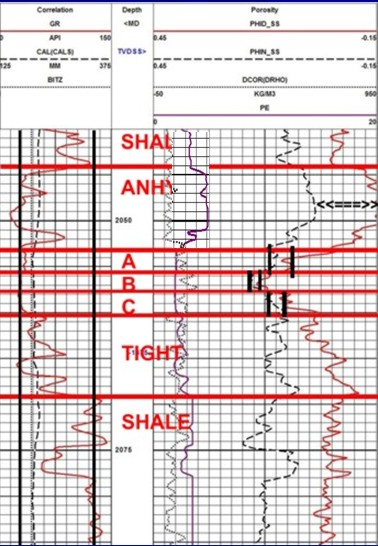Example of a gamma ray log (solid black curve in Track 1) forming the correlation curve on a density neutron log. The geologists picks for the clean sand and pure shale lines are the two vertical black lines in Track 1. Bed boundaries and overall lithology are interpreted from the response of all the log curves.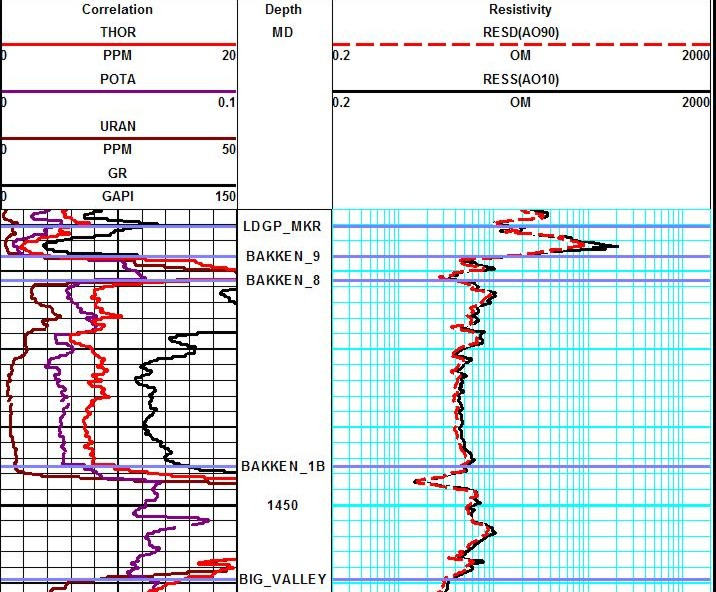Example of a spectral gamma ray log (Track 1) on a resistivity log on low resistivity, radioactive Bakken sand (4 ohm-m in best sand). Note high resistivity upper and lower shales, which are the source rock for the oil in the sand. These are "real" shales with gamma ray readings between 250 and 500 API units. Spectral GR shows low but significant uranium content in sand and very high uranium in the shales, associated with the kerogen content. The thorium curve is the best clay indicator.

Page Views ---- Since 01 Jan 2015
Copyright 2023 by Accessible Petrophysics Ltd.
CPH Logo, "CPH", "CPH Gold Member", "CPH Platinum Member", "Crain's Rules", "Meta/Log", "Computer-Ready-Math", "Petro/Fusion Scripts" are Trademarks of the Author